Function Repository Resource:

# RoundedPercentageAllocation

Split a value into list of rounded numbers according to a list of target proportions

Contributed by: Jon McLoone
 ResourceFunction["RoundedPercentageAllocation"][total,proportions] creates a list of integers that sum to total and are also as close to the given proportions as possible. ResourceFunction["RoundedPercentageAllocation"][total,proportions,d] creates a list of numbers that sum to total and are multiples of d.

## Examples

### Basic Examples (2)

Split 100 into proportions of 1/3 and 2/3 to the nearest integer:

 In:=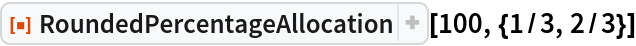Out=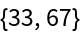In most financial currencies, one would typically allocate it to the nearest 0.01:

 In:=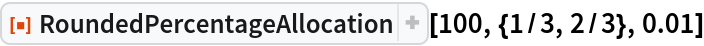Out=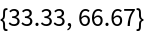### Properties and Relations (2)

As long as the total is a multiple of the allocation amount, the result will always sum to the original total:

 In:=Out=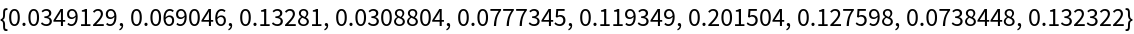In:=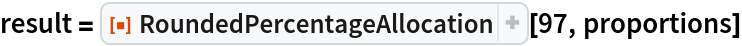Out=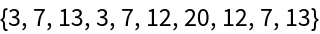In:=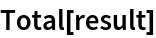Out=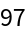In:=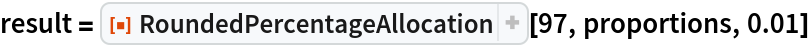Out=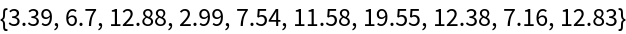In:=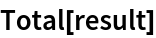Out=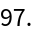However, the proportions in the result are usually not exactly the requested proportions:

 In:=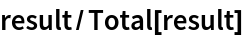Out=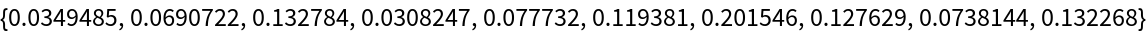### Possible Issues (2)

If the input number is not divisible by the allocation size then the result will add up to less then the original:

 In:=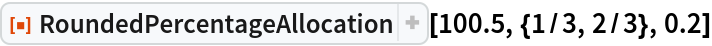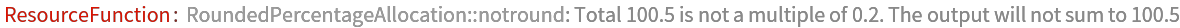Out=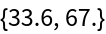In:=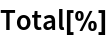Out=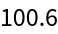If you allocate an amount to proportions that do not sum to one, the proportions will be rescaled so that they do sum to 1:

 In:=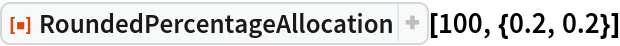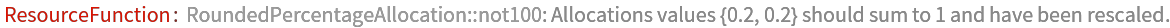Out=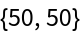Jon McLoone

## Version History

• 1.0.0 – 05 February 2020